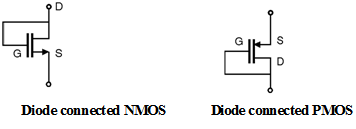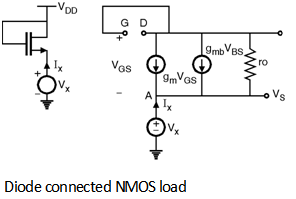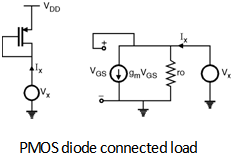Home > Analog CMOS Design > MOSFET Amplifiers > CS Amplifier with Active Load

CS Amplifier with Active Load :

A MOSFET can operate as a resistor if its gate and drain terminals are shorted. This type of configuration is called as â€œdiode connectedâ€ resistor as shown in Figure below.In CMOS technology it is difficult to fabricate resistors with tightly controlled values of physical size. Hence the load resistor RD is replaced by the MOS transistor.

To find the load resistance of the diode connected NMOS load consider the circuit shown in
Figure below and its corresponding small signal equivalent circuit.By applying KVL,.

We have, VGS = VG - VS = - Vx

VBS = VB - VS = - Vx [ Body is connected to ground]

By applying KCL at Node A,

We get,

Ix + gm VGS + gmb V BS + = 0

i.e. Ix = gm Vx + gmb V x + = Vx

=

i.e. = = || ro

\ The load resistance of NMOS diode connected load is given by || ro

For PMOS diode connected load consider Figure shown below, which shows the circuit to calculate load resistance and its small signal equivalent circuit.Now, we have VGS = Vx

\ By applying KCL,

We get, Ix = gm VGS + = gm V x +

Ix = Vx

= = = || ro

\ The load resistance of PMOS diode connected load is given as || ro.

Now consider the CS amplifier with diode connected load shown in figure below.Here the gain of the amplifier is given by replacing the RD with the corresponding load resistance of NMOS and PMOS diode connected loads.

\ For NMOS diode connected load.

An = - gm1